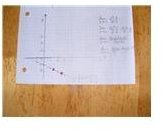# A Guide to Understanding Calculus Limit Problems

Page content

## Introducing Limits

Students learning calculus limit problems need to know what a limit of a function is before they can evaluate them. The concept of

limits is finding the value of a function as x approaches a certain value, a. For example, in the linear function y = 3x-5, as x approaches 2, y takes on the value of 1. This is solved by substituting 2 into the function:

lim y = 3x – 5 = 3(2) - 5 = 6 - 5 = 1

y→2

Students should be shown graphs of the functions so they can understand the concept of limit. Limits may or may not be continuous at the value a in a graph. Textbooks are good sources of graphs showing continuous and discontinuous functions. If you have a smartboard you may want to try an online interactive gallery for graphing functions which can be found at: Maths Online from the University of Vienna, Austria. The limit theory should explain the limit of afunction as x approaches from the left and right.

For example, in the function:

lim y = x2- 4, the function does not exist as the value x approaches 2, but the limit exists.

x→2 2 –x

The limit exists as the graph approaches from the left side and right side of the function. From the left and right side of the limiting x value 2, the y value is approaching -4.

## Evaluating Limits

There are several ways to solve limits. Limits can be solved by the substitution method. This is the easiest method and should be tried first. If the result of direct substitution results in a function that is 0/0, the answer is undefined at this point, yet the limit still exists. To evaluate the limits students need to be shown several examples. Limits that need simplification need to be factored or multiplied by the conjugate.

Examples of solving these types of limits are found in the study guide on How to Solve Calculus Limit Problems.

Students then can be shown more complicated forms of a function. Solving these types of limits involves factoring of the difference of squares, factoring special cases with square roots in the numerator and denominator, as well as factoring polynomials. For reminders on factoring squares and cubes review these algebraic concepts at: PurpleMaths: Special Factoring of Squares and Cubes.

Examples of solving special cases of Calculus limit problems are found in the study guide on How to Solve Special Cases of Calculus Limit Problems.

Teachers should demonstrate how to solve samples of all of the types. Practice sheets with many problems will reinforce the concept. Review the answers and related graphs before continuing. Practice at graphing functions that approach a value from the left and right are not necessary when evaluating Calculus limit problems.

## Calculus Resources

An online video explaining both the graphs and the concept of limits can be found at Tutorials for the Calculus Phobe. Showing a video can simplify and provide another explanation for students since limits are a new concept for them. Explanations from textbooks can be very theoretical. This series of videos is easy to understand.

Many other tutorials, books, study guides and help with problems can be found on the Internet.

Teachers will find interactive resources, online videos, movies, games, worksheets, activities and formulas as resources for pre-calculus at: Mathbits.

Students who are provided with many examples and time to practice Calculus limit problems will find an understanding of the limit concept easy. High school students will then begin to appreciate how Calculus theory can be applied to practical problems in the real world.

• Photo Credits: Lila Kallstrom